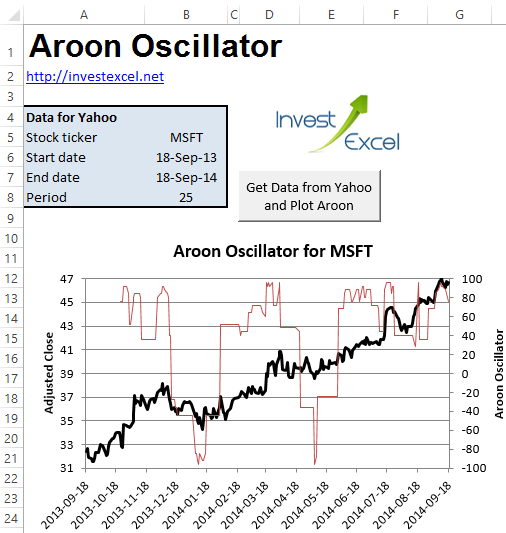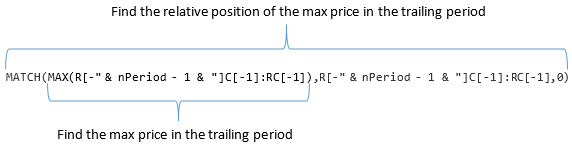Calculate Aroon with Excel and VBA

This is the second in a two-part article about the Aroon indicator.

This part introduces a Excel spreadsheet that plots the indicator via data supplied via connectivity to Yahoo Finance. VBA automates the entire process.

You can download the complete spreadsheet from the link at the bottom of this post. However, read the rest of this article to learn how the critical parts of the VBA are structured.Briefly, Aroon consists of three signals

• Aroon up  is equal to 100 x (period – number of days since period high) / period
• Aroon down is equal to 100 x (period – number of days since period low) / period
• Aroon oscillator is equal to Aroon up minus Aroon down.

The period is usually 25 days, but can be made longer or shorter to quantify the strength of trends with different characteristic time periods.

The VBA contains two major subroutines.

• The second calculates Aroon, and creates two plots – one for the oscillator (and the close price), and another for the up/down signals

The most important part of the Aroon subroutine are the following lines

 dataSheet.Range("h" & 1 + nperiod & ":h" & nRows).FormulaR1C1 = _ "=100-100*(1" & "-MATCH(MAX(R[-" & nPeriod - 1 & "]C[-1]:RC[-1]),R[-" & nPeriod - 1 & "]C[-1]:RC[-1],0)/" & nPeriod & ")"   dataSheet.Range("i" & 1 + nperiod & ":i" & nRows).FormulaR1C1 = _ "=100-100*(1" & "-MATCH(MIN(R[-" & nPeriod - 1 & "]C[-2]:RC[-2]),R[-" & nPeriod - 1 & "]C[-2]:RC[-2],0)/" & nPeriod & ")"   dataSheet.Range("j" & 1 + nPeriod & ":j" & nRows).FormulaR1C1 = _ "=RC[-2]-RC[-1]"

The adjusted close prices are in column h (in the sheet dataSheet), nRows is the number of rows, and nPeriod is the number of  rows of historical data.The first line calculates Aroon up, the second Aroon down, and the third the oscillator.

The VBA use the R1C1 cell referencing method to programmatically create the formulas. For the up and down code,

• Max() and Min() calculate the maximum or minimum price in the trailing time period
• Match() calculates the position of the maximum or minimum price in the trailing time period

This, for example, is the structure of the nested Match/Max command for Aroon up (where R[-” & nPeriod – 1 & “]C[-1]:RC[-1] is the Excel range of close prices in the trailing time period)This is the entire VBA for the Aroon function, including the code that creates the plots.

 Sub Aroon()   Set parameterSheet = Sheets("Parameters") Set dataSheet = Sheets("Data")   nRows = dataSheet.UsedRange.Rows.Count - 1 nPeriod = parameterSheet.Range("nPeriod")   dataSheet.Range("h1") = "Aroon Up" dataSheet.Range("i1") = "Aroon Down" dataSheet.Range("j1") = "Aroon Oscillator"   dataSheet.Range("h" & 1 + nPeriod & ":h" & nRows).FormulaR1C1 = _ "=100-100*(1" & "-MATCH(MAX(R[-" & nPeriod - 1 & "]C[-1]:RC[-1]),R[-" & nPeriod - 1 & "]C[-1]:RC[-1],0)/" & nPeriod & ")"   dataSheet.Range("i" & 1 + nPeriod & ":i" & nRows).FormulaR1C1 = _ "=100-100*(1" & "-MATCH(MIN(R[-" & nPeriod - 1 & "]C[-2]:RC[-2]),R[-" & nPeriod - 1 & "]C[-2]:RC[-2],0)/" & nPeriod & ")"   dataSheet.Range("j" & 1 + nPeriod & ":j" & nRows).FormulaR1C1 = _ "=RC[-2]-RC[-1]"   Set parameterSheet = parameterSheet Set dataSheet = dataSheet   Dim ch As ChartObject   For Each ch In parameterSheet.ChartObjects ch.Delete Next   Dim AroonChart As ChartObject Set AroonChart = parameterSheet.ChartObjects.Add(Left:=Range("a11").Left, Width:=500, Top:=Range("a11").Top, Height:=300)   With AroonChart.Chart   .Parent.Name = "AroonOscillator"   With .SeriesCollection.NewSeries .ChartType = xlLine .AxisGroup = xlPrimary .XValues = dataSheet.Range("a2:a" & nRows) .Values = dataSheet.Range("g2:g" & nRows) .Name = "Adjusted Close" .Border.ColorIndex = 1 .Format.Line.Weight = 2 End With   With .SeriesCollection.NewSeries   .ChartType = xlLine .XValues = dataSheet.Range("a2:a" & nRows) .Values = dataSheet.Range("j2:j" & nRows) .Format.Line.Weight = 1 .Name = "Aroon Oscillator" .AxisGroup = xlSecondary   End With   .Axes(xlValue, xlPrimary).HasTitle = True .Axes(xlValue, xlPrimary).AxisTitle.Characters.Text = "Adjusted Close" .Axes(xlValue, xlSecondary).HasTitle = True .Axes(xlValue, xlSecondary).AxisTitle.Characters.Text = "Aroon Oscillator" .Axes(xlValue, xlSecondary).MaximumScale = 100 .Axes(xlValue, xlSecondary).MinimumScale = -100 .Axes(xlValue, xlPrimary).MaximumScale = WorksheetFunction.Max(dataSheet.Range("g2:g" & nRows)) .Axes(xlValue, xlPrimary).MinimumScale = Int(WorksheetFunction.Min(dataSheet.Range("g2:g" & nRows))) .Legend.Position = xlLegendPositionBottom .SetElement (msoElementChartTitleAboveChart) .ChartTitle.Text = "Aroon Oscillator for " & parameterSheet.Range("ticker") .ChartTitle.Font.Size = 14   End With   Dim AroonUpDownChart As ChartObject Set AroonUpDownChart = parameterSheet.ChartObjects.Add(Left:=Range("a32").Left, Width:=500, Top:=Range("a32").Top, Height:=300)   With AroonUpDownChart.Chart   .Parent.Name = "Aroon Up & Dow"   With .SeriesCollection.NewSeries .ChartType = xlLine .AxisGroup = xlPrimary .XValues = dataSheet.Range("a2:a" & nRows) .Values = dataSheet.Range("h2:h" & nRows) .Name = "Up" .Border.ColorIndex = 1 .Format.Line.Weight = 2 .Border.Color = RGB(119, 158, 203)   End With   With .SeriesCollection.NewSeries   .ChartType = xlLine .XValues = dataSheet.Range("a2:a" & nRows) .Values = dataSheet.Range("i2:i" & nRows) .Format.Line.Weight = 2 .Name = "Down" .AxisGroup = xlPrimary .Border.Color = RGB(222, 165, 164)   End With   .Axes(xlValue, xlPrimary).HasTitle = True .Axes(xlValue, xlPrimary).AxisTitle.Characters.Text = "Aroon Up & Down Trend" .Axes(xlValue, xlPrimary).MaximumScale = 100 .Axes(xlValue, xlPrimary).MinimumScale = 0 .Legend.Position = xlLegendPositionBottom .SetElement (msoElementChartTitleAboveChart) .ChartTitle.Text = "Aroon Up & Down for " & parameterSheet.Range("ticker") .ChartTitle.Font.Size = 14   End With   End Sub

The spreadsheet is simple to use, and contains the code above. You just enter a ticker, two dates and click a button. Feel free to learn, modify and expand the VBA.